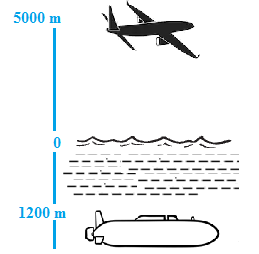# Ex.1.1 Q4 Integers - NCERT Maths Class 7

Go back to  'Ex.1.1'

## Question

A plane is flying $$5000\rm\,m$$ above the sea level. At a particular point, it is exactly above a submarine floating $$1200\rm\,m$$  below the sea level. What is the vertical distance between them?Video Solution
Integers
Ex 1.1 | Question 4

## Text Solution

What is known?

Distance of plane and submarine from the sea level

What is unknown?

The vertical distance between plane and submarine.

Steps:

Height of the plane above the sea level $$= 5000 \rm\,m$$

Depth of submarine below the sea level $$= \,–1200 \rm\,m$$

Distance between plane and submarine

\begin{align}& = 5000\rm{}\,\, m -\left( \rm{}-1200\, m \right)\\\rm{} &= 5000 + 1200\\& = 6200\rm{} m\\{\rm{}}\end{align}

Hence, the vertical distance between $$\rm{}= 6200m.$$

Learn from the best math teachers and top your exams

• Live one on one classroom and doubt clearing
• Practice worksheets in and after class for conceptual clarity
• Personalized curriculum to keep up with school